PB 1.

LO 5.3The following product costs are available for Stellis Company on the production of erasers: direct materials, $22,000; direct labor,$35,000; manufacturing overhead, $17,500; selling expenses,$17,600; and administrative expenses; $13,400. 1. What are the prime costs? 2. What are the conversion costs? 3. What is the total product cost? 4. What is the total period cost? 5. If 13,750 equivalent units are produced, what is the equivalent material cost per unit? 6. If 17,500 equivalent units are produced, what is the equivalent conversion cost per unit? PB 2. LO 5.3The following product costs are available for Kellee Company on the production of eyeglass frames: direct materials,$32,125; direct labor, $23.50; manufacturing overhead, applied at 225% of direct labor cost; selling expenses,$22,225; and administrative expenses, $31,125. The direct labor hours worked for the month are 3,200 hours. 1. What are the prime costs? 2. What are the conversion costs? 3. What is the total product cost? 4. What is the total period cost? 5. If 6,425 equivalent units are produced, what is the equivalent material cost per unit? 6. What is the equivalent conversion cost per unit? PB 3. LO 5.3Vexar manufactures nails. Manufacturing is a one-step process where the nails are forged. This is the information related to this year’s production: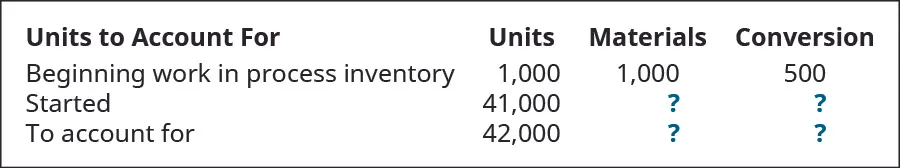Ending inventory was 100% complete as to materials and 70% complete as to conversion, and the total materials cost is$115,080 and the total conversion cost is $72,072. Using the weighted-average method, what are the unit costs if the company transferred out 34,000 units? Using the weighted-average method, prepare the company’s process cost summary for the month. PB 4. LO 5.3During March, the following costs were charged to the manufacturing department:$22,500 for materials; $45,625 for labor; and$50,000 for manufacturing overhead. The records show that 40,000 units were completed and transferred, while 10,000 remained in ending inventory. There were 45,000 equivalent units of material and 42,500 units of conversion costs. Using the weighted-average method, prepare the company’s process cost summary for the month.

PB 5.

LO 5.3Ardt-Barger has a beginning work in process inventory of 5,500 units and transferred in 25,000 units before ending the month with 3,000 units that were 100% complete with regard to materials and 80% complete with regard to conversion costs. The cost per unit of material is $5.45, and the cost per unit for conversion is$6.20 per unit. Using the weighted-average method, prepare the company’s process cost summary for the month.

PB 6.

LO 5.3The following data show the units in beginning work in process inventory, the number of units started, the number of units transferred, and the percent completion of the ending work in process for conversion. Given that materials are added at the beginning of the process, what are the equivalent units for material and conversion costs for each quarter using the weighted-average method? Assume that the quarters are independent.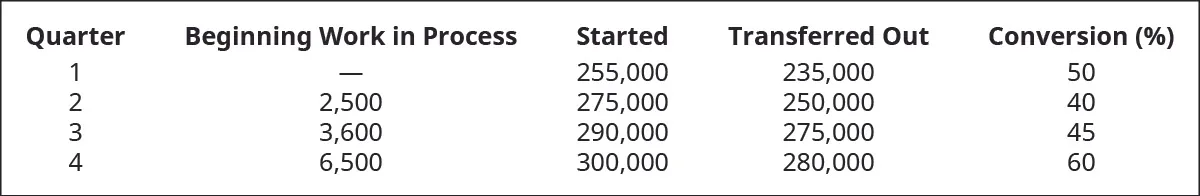PB 7.

LO 5.3The following data show the units in beginning work in process inventory, the number of units started, the number of units transferred, and the percent completion of the ending work in process for conversion. Given that materials are added 50% at the beginning of the process and 50% at the end of the process, what are the equivalent units for material and conversion costs for each quarter using the weighted-average method? Assume that the quarters are independent.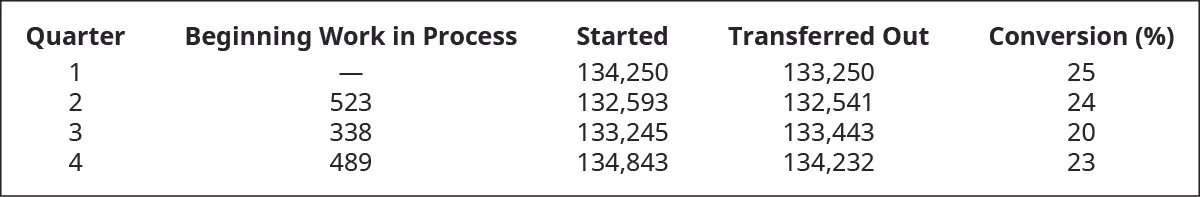PB 8.

LO 5.3The following data show the units in beginning work in process inventory, the number of units started, the number of units transferred, and the percent completion of the ending work in process for conversion. Given that materials are added 50% at the beginning of the process and 50% at the end of the process, what are the equivalent units for material and conversion costs for each quarter using the weighted-average method? Assume that the quarters are independent.PB 9.

LO 5.4The finishing department started the month with 600 units in WIP inventory. It received 1,500 units from the molding department and ended the month with 550 units still in process. How many units were transferred out?

PB 10.

LO 5.4The packaging department began the month with 750 units that were 100% complete with regard to material and 25% complete with regard to conversion. It received 9,500 units from the processing department and ended the month with 500 units that were 100% complete with regard to materials and 75% complete with regard to conversion. With a $7 per unit material cost and a$4 per unit cost for conversion, what is the cost of the units transferred out and remaining in ending inventory?

PB 11.

LO 5.4Production information shows these costs and units for the smoothing department in August.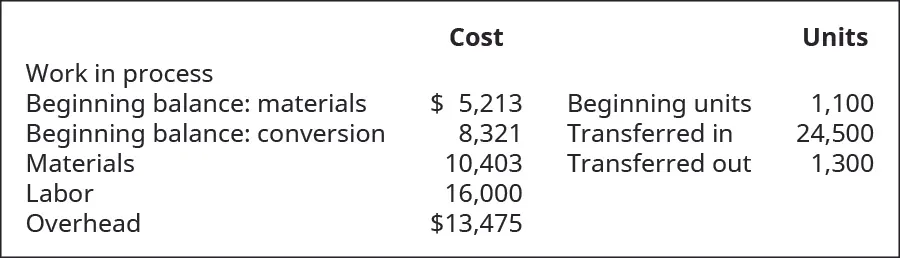What is the value of the inventory transferred out to finished goods and the value of the WIP inventory at the end of the month, assuming conversion costs are 30% complete?

PB 12.

LO 5.4Given the following information, prepare a production report with materials added at the beginning and ending work in process inventory being 80% complete with regard to conversion costs.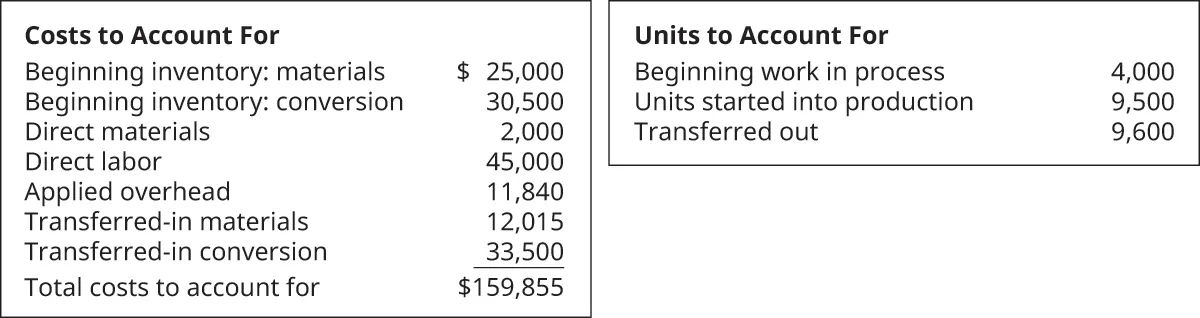PB 13.

LO 5.5Selected information from Hernandez Corporation shows the following: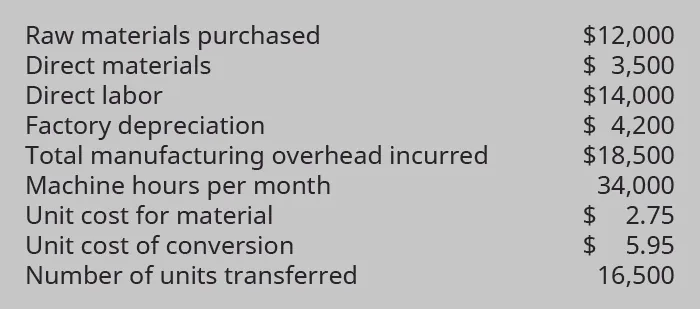Prepare journal entries to record the following:

1. raw material purchased
2. direct labor incurred
3. depreciation expense (hint: this is part of manufacturing overhead)
4. raw materials used
5. overhead applied on the basis of $0.50 per machine hour 6. the transfer from department 1 to department 2 PB 14. LO 5.5Rexar had 1,000 units in beginning inventory before starting 9,500 units and completing 8,000 units. The beginning work in process inventory consisted of$5,000 in materials and $8,500 in conversion costs before$16,000 of materials and \$18,500 of conversion costs were added during the month. The ending WIP inventory was 100% complete with regard to materials and 40% complete with regard to conversion costs. Prepare the journal entry to record the transfer of inventory from the manufacturing department to the finished goods department.

Order a print copy

As an Amazon Associate we earn from qualifying purchases.